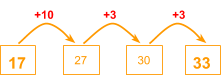# Subtraction

Go back to  'Addition & Subtraction'

## Introduction to Subtraction

Imagine there is a stack of 3 oranges on the table. You take one out, well now there are two. Conceptually, what you have done is taken a smaller group out of a larger group and that results in the original group of objects becoming smaller.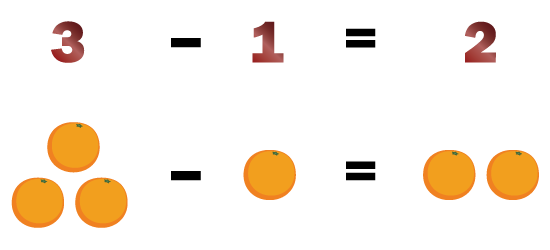That is why subtraction is a very basic operation that we need every day in our lives. We will try to understand the process of subtraction today.

## The Big Idea: Subtraction

### A simple idea: What is subtraction?

The act of taking away a certain number of objects from a collection of objects is called subtraction. When there are 25 people travelling in a bus, and 3 people get down at a bus stop, then we need to subtract 3 from 25 to understand how many people are still in the bus.

25 – 3 = 22

You can consider subtraction to be the opposite of addition. In addition you keep on putting together objects to get a larger group of objects, but subtraction involves taking away objects from a larger group of objects.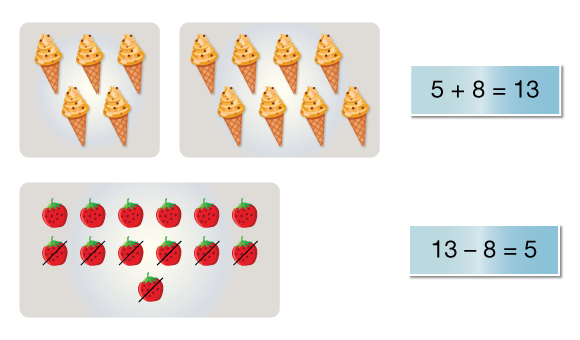A simple idea used for subtraction of two numbers is the place value method. Let us say you need to subtract 234 from 1568. In order to correctly subtract them, you need to be clear about what are the place values of these two numbers. This is how they would be written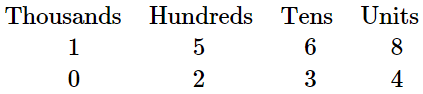So, in order to subtract 234 from 1568, you write both the numbers one below the other in such a way that the place values are all aligned. So, it would look like this:

\begin{align} {\rm{1}}\;\;{\rm{5}}\;\;{\rm{6}}\;\;{\rm{8}}\\ {\rm{ - }}\;\,{\rm{2}}\;\;{\rm{3}}\;\;{\rm{4}}\\ \overline {\underline {1\;\;3\;\;3\;\;4} } \end{align}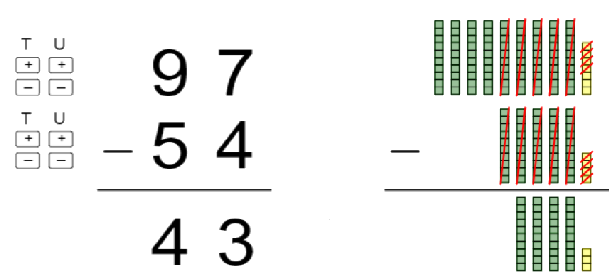## How is it important?

### The concept of borrowing

When we subtracted 234 from 1568, the corresponding numbers in each place was bigger for the larger number than the smaller number. For the unit’s place, 8 was bigger than 4, for the tens place, 6 was bigger than 3, and for hundreds place 5 was bigger than 2. That is why we were able to subtract in a simple manner. Even in the widget shown above, when 54 was subtracted from 97, 7 was bigger than 4 in the Unit’s place and 9 was bigger than 5 in the tens place.

But what if we had to subtract 268 from 1534? In that case, the numbers would be written in this way

\begin{align} {\rm{1}}\;\;{\rm{5}}\;\;{\rm{3}}\;\;{\rm{4}}\\ {\rm{ - }}\;\,{\rm{2}}\;\;{\rm{6}}\;\;{\rm{8}}\\ \overline {\underline {\qquad\quad\;\;\,\\} } \end{align}

Would you be able to subtract 8 from 4? No.
Would you be able to subtract 6 from 3? No

So, what would be the solution? The solution would be to ‘borrow’ from the neighbour. What this means is that when this happens in the unit’s place, you need to borrow from the tens place. And if it happens in the tens place, the borrowing would be from the hundred’s place.

2 1 4
1 5 3 4
– 2 6 8
6

As you can see above, a ten has been borrowed from the 3 tens we have, so the tens place becomes 2, and the 4 in the unit’s place becomes 14. Now 8 can be subtracted from 14 to give 6 which we write down as the first step.

Next, we see that in the tens place 6 can’t be subtracted from 2, so we borrow one from the 5 in hundreds place, and now this is how it would look

12
4 2 1 4
5 3 4
– 2 6 8
6 6

Now, we can subtract 6 from 12, and can put the answer 6 in the tens place. Now we see that in the hundred’s place it is possible to subtract 2 from 4 and no more borrowing is needed. Also, in the thousand’s place it is possible to subtract 0 from 1. This gives 1266 as the answer.

12
4 2 1 4
5 3 4
– 2 6 8
1 2 6 6

## Common mistakes or misconceptions

• When solving 803 - 65, children first have to subtract 5 from 3. Instead of borrowing from higher place value, they simply subtract 3 from 5 and write down 2 in the ones place. This confusion comes because when adding numbers, we say the order does not matter. But the same does not apply when subtracting. Modelling the subtraction using Base-10 blocks helps address the misconception.
• While subtracting, if students have to borrow from a higher place value where the digit is zero, children make mistakes. This is because they have to keep going to higher and higher place values until there is a non-zero digit for them to borrow from.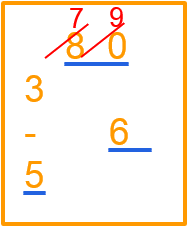Additional targeted practice of similar questions helps avoid this mistake.

• When subtracting a three-digit number to a two-digit number, students may place the numbers one below the other incorrectly.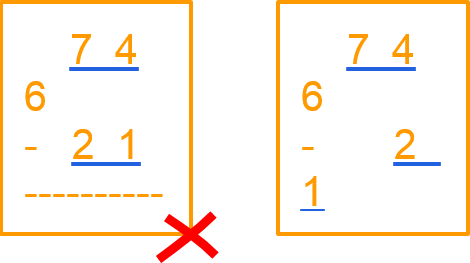This generally happens once again due to an incomplete understanding of place values and the idea of addition. Rather than telling students to simply align numbers from the right, it is better to ask them to model this addition problem using Base-10 blocks, so they understand why the numbers are aligned from the right.

## Tips and Tricks

• Help children associate subtraction with common words used in everyday language like “difference”, “less than”, “more than”, “how much more”, “left with”, “remain”, etc. This association will help them with comprehending word problems.
• When subtracting mentally, sometimes counting up from the smaller number to the larger number can be a useful strategy to find the difference. E.g. to find the difference between 33 and 17, it may be easier to start with 17 and see how much we need to add to get to 33. Doing this in steps allows us to mentally calculate the difference as 16.